LearnFast.Ninja

# Calculate percentages in Excel 2010

In this tutorial we will show you how to calculate percentage in Excel 2010. In this example we have number of students and number of students who pass the exam. In the Success rate cell we calculate the percentage of students that pass the exam.

Step 1: Build this table.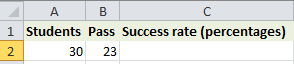Step 2: Write this formula =(B2/A2). Divide the smallest with the largest.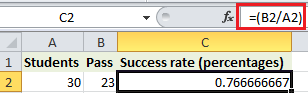Step 3: Right click the cell then click Format Cells.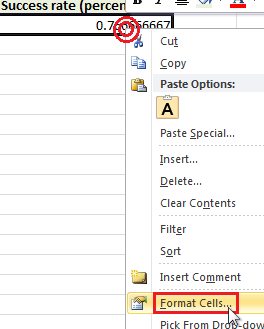Step 4: In Number tab click Percentage then change the Decimal places to 0 and click OK.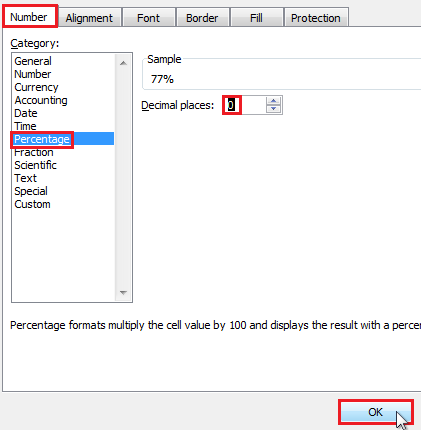Result: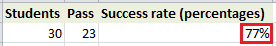Tags

excel